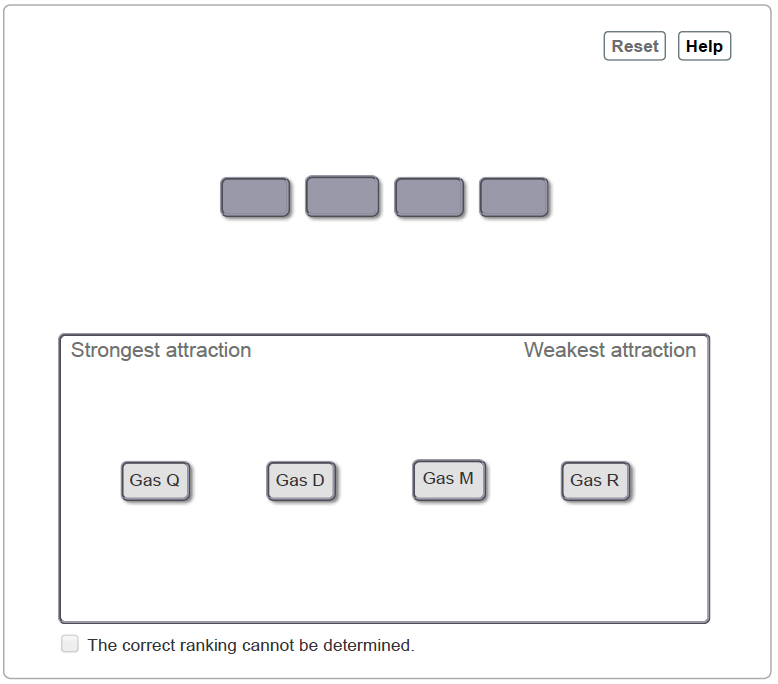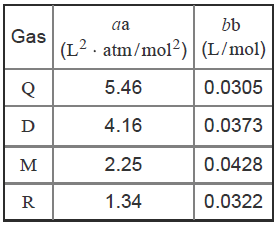# Problem: Based on the given van der Waals constants, arrange these hypothetical gases in order of decreasing strength of intermolecular forces. Assume that the gases have similar molar masses.Rank from strongest to weakest intermolecular attraction. To rank items as equivalent, overlap them.You may be familiar with the ideal gas equation,PV=nRTwhere n is the number of moles, P is the pressure in atmospheres, V is the volume in liters, and R is equal to 0.08206 L ⋅ atm/(mol ⋅ K).Real gases, especially under extremes of temperature and pressure, deviate from ideal behavior due to intermolecular forces and the volume occupied by the gas molecules themselves. Correction factors can be applied to the ideal gas equation to arrive at the van der Waals equation,P=nRTV-nb-n2aV2where a and b are van der Waals constants for the particular gas. The term nb corrects the volume to account for the size of the molecules and the term n2a/V2 corrects the pressure for intermolecular attraction.van der Waals constants for hypothetical gases

###### FREE Expert Solution
95% (102 ratings)View Complete Written Solution
###### Problem Details

Based on the given van der Waals constants, arrange these hypothetical gases in order of decreasing strength of intermolecular forces. Assume that the gases have similar molar masses.

Rank from strongest to weakest intermolecular attraction. To rank items as equivalent, overlap them.You may be familiar with the ideal gas equation,

$\mathbf{PV}\mathbf{=}\mathbf{nRT}$

where n is the number of moles, P is the pressure in atmospheres, V is the volume in liters, and R is equal to 0.08206 L ⋅ atm/(mol ⋅ K).

Real gases, especially under extremes of temperature and pressure, deviate from ideal behavior due to intermolecular forces and the volume occupied by the gas molecules themselves. Correction factors can be applied to the ideal gas equation to arrive at the van der Waals equation,

$\mathbf{P}\mathbf{=}\frac{\mathbf{nRT}}{\mathbf{V}\mathbf{-}\mathbf{nb}}\mathbf{-}\frac{{\mathbf{n}}^{\mathbf{2}}\mathbf{a}}{{\mathbf{V}}^{\mathbf{2}}}$

where a and b are van der Waals constants for the particular gas. The term nb corrects the volume to account for the size of the molecules and the term n2a/V2 corrects the pressure for intermolecular attraction.

van der Waals constants for hypothetical gases